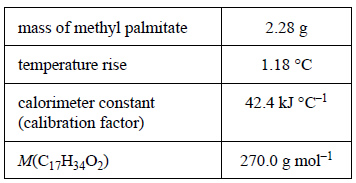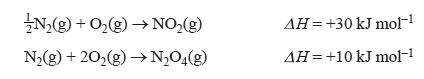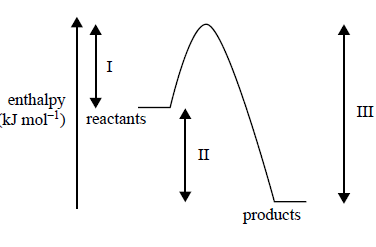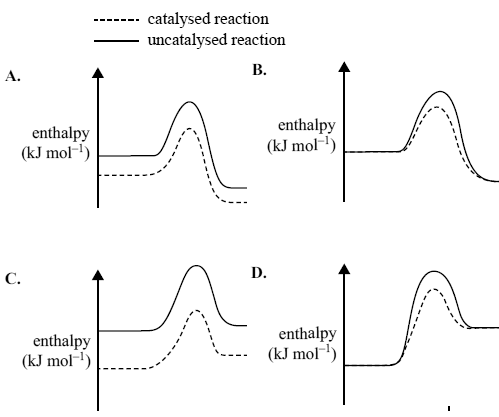Enthalpy (2015 VCE) 1) A weighed sample of methyl palmitate, C17H34O2, was burnt in excess oxygen in a bomb calorimeter. The experimental results are shown in the following table.a) Use the data provided to calculate the molar enthalpy of combustion of the methyl palmitate Solutionb) Write a balanced thermochemical equation for the combustion reaction. Solution2) Consider the reaction shown in the following equation.The enthalpy change for the reaction N2O4(g) → 2NO2(g) is A. –50 kJ mol–1 B. +20 kJ mol–1 C. +50 kJ mol–1 D. +70 kJ mol–1 Solution3) Consider the following energy profi le for a particular chemical reaction, where I, II and III represent enthalpy changes during the reaction. Which one of the following statements is correct? A. The activation energy for the reverse reaction is (III–II). B. The net energy released for the forward reaction is represented by II. C. The energy required to break the reactant bonds is represented by II. D. The energy released by the formation of new bonds is represented by I. Solution4) The oxidation of sulfur dioxide is an exothermic reaction. The reaction is catalysed by vanadium(V) oxide. 2SO2(g) + O2(g) -> 2SO3(g) Which one of the following energy profile diagrams correctly represents both the catalysed and the uncatalysed reaction?Solution5) Consider the following equations. 1/2 N2(g) + O2(g) → NO2(g) H = +30 kJ mol–1 N2(g) + 2O2(g) → N2O4(g) H = +10 kJ mol–1 The enthalpy change for the reaction N2O4(g) → 2NO2(g) is A. –50 kJ mol– B. +20 kJ mol– C. +50 kJ mol– D. +70 kJ mol– Solution